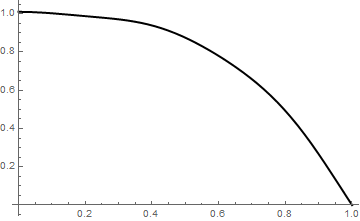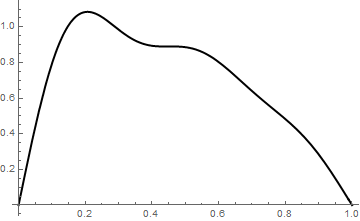# Bessel Expansions

This section presents Bessel's expansions for functions defined on a finite interval (0,ℓ). This expansions has similar properties as Fourier series, including Gibbs phenomenon.

Introduction to Linear Algebra with Mathematica

# Bessel functions

Bessel's equation of order $$\nu$$

$x^2 y'' + x\,y' + \left( x^2 - \nu^2 \right) y =0 \qquad\mbox{or in self-adjoint form}\qquad \frac{\text d}{{\text d}x} \left( x\,\frac{{\text d} y}{{\text d} x} \right) + \left( x - \frac{\nu^2}{x}\right) =0$
has a regular singularity at x = 0. This differential equation together with boundary condition
$a\,y(\ell ) + b\,y' (\ell ) =0$
becomes a singular Sturm--Liouville problem that has eigenfunctions expressed through Bessel functions. The substitution $$y =u x^{-1/2}$$ yields the reduced form of Bessel's equation
$u'' + \left( 1 + \frac{1- 4\nu^2}{4x^2} \right) u=0 .$
This equation was first solved by Daniel Bernoulli (1700-1782), the second son of Johann Bernoulli, who studied the oscillations of a chain suspended by one end, and Leonhard Euler (1707--1783), who analyzed the vibrations of a stretched membrane. However, the systematic analysis of solutions to the above equation was conducted around 1817 by the German astronomer Friedrich Wilhelm Bessel (1784--1846) during an investigation of solutions of one of Kepler’s equations of planetary motion.

The coefficients of the Frobenius solution

$y(x) = \sum_{k\ge 0} a_k x^{k+\sigma}$
satisfy
$\sum_{k\ge 0} a_k \left[ (k+\sigma )^2 - \nu^2 \right] x^{k+\sigma} + \sum_{k\ge 2} a_{k-2} x^{k+\sigma} =0.$
The indicial equation $$\sigma^2 = \nu^2$$ has two roots $$\sigma = \pm \nu$$ to which correspond two linearly independent solutions.

The solution that is finite at x = 0 can be represented by

$J_{\nu} (x) = \left( \frac{x}{2} \right)^{\nu} \sum_{k\ge 0} \frac{(-1)^k x^{2k}}{4^k k! \Gamma (k+\nu +1)} ,$
where Γ is the Gamma function of Euler. The above series (which converges for all real or complex x) defines the Bessel function of the first kind. If $$\nu$$ is not an integer, then the function $$J_{-\nu} (x)$$ is linearly independent of $$J_{\nu} (x) .$$ Therefore these two functions constitute the fundamental set of solutions to Bessel's equation. When $$\nu =n$$ is an integer,
$J_{-n} (x) = (-1)^n \,J_{n} (x) ,$
and we need to find another linearly independent solution to Bessel's equation. This can be done using reduction of order, which leads to another linearly independent solution:
$J_{n} (x) \,\int \frac{{\text d} x}{x \left[ J_n (x) \right]^2} .$
Upon multiplication by a suitable constant, this expression defines either $$Y_n (x) = \pi N_n (x),$$ the Weber function, or $$N_n (x) ,$$ the Neumann function. Heinrich Martin Weber (1842--1913) and Carl (also Karl) Gottfried Neumann (1832--1925) were German mathematicians. The Neumann function can be defined by a single expression:
$N_{\nu} (x) = \frac{\cos \nu\pi \,J_{\nu} (x) - J_{-\nu} (x)}{\sin \nu\pi} ,$
which is valid for all values of $$\nu .$$ If $$\nu =n ,$$ an integer, then l'Hopital's rule yields
\begin{align*} Y_n (x) &= \pi\,N_n (x) = 2\,J_n (x) \left( \ln \frac{x}{2} + \gamma \right) - \sum_{k=0}^{n-1} \frac{(n-k-1)!}{k!} \left( \frac{x}{2} \right)^{2k-n} \\ &\quad - \sum_{k\ge 0} \frac{(-1)^k x^{2k+n}}{2^{2k+n} k! (n+k)!} \left[ 2 \left( 1 + \frac{1}{2} + \frac{1}{3} + \cdots + \frac{1}{n} \right) + \frac{1}{k+1} + \frac{1}{k+2} + \cdots + \frac{1}{k+n} \right] , \end{align*}
where $$\gamma = \Gamma '(1) \approx 0.5772156 \ldots$$ is the Euler constant. Bessel functions can be expressed through the single Bessel function of order zero:
$J_n (x) = {\bf j}^n T_n \left( {\bf j}\,\frac{\text d}{{\text d}x} \right) J_0 (x) , \qquad n=1,2,\ldots ,$
where Tn is the Chebychev polynomial of the first kind and j is the unit vector in positive vertical direction on the complex plane. The parametric Bessel differential equation
$x^2 y'' + x\,y' + \left( \alpha^2 x^2 - \nu^2 \right) y =0$
has the general solution on $$(0, \infty ) :$$
$y(x) = A\,J_{\nu} (\alpha x ) + B\, Y_{\nu} (\alpha x ) \qquad \mbox{or} \qquad y(x) = A\,J_{\nu} (\alpha x ) + B\, N_{\nu} (\alpha x ) ,$
with arbitrary constants A and B. When $$\alpha = {\bf j} ,$$ the imaginary unit ($${\bf j}^2 =-1$$ ), we get the modified Bessel equation
$x^2 y'' + x\,y' - \left( x^2 + \nu^2 \right) y =0 \qquad\mbox{or in self-adjoint form}\qquad \frac{\text d}{{\text d}x} \left( x\,\frac{{\text d} y}{{\text d} x} \right) - \left( x + \frac{\nu^2}{x}\right) =0$
that has two linearly independent solutions:
\begin{align*} I_{\nu} (x) &= {\bf j}^{-\nu} \,J_{\nu} \left( {\bf j} x \right) = \sum_{k\ge 0} \frac{1}{k! \,\Gamma (k + \nu +1)} \left( \frac{x}{2} \right)^{2k+\nu} , \\ K_{\nu} (x) &= \frac{\pi}{2} \,\frac{I_{-\nu}(x) - I_{\nu} (x)}{\sin \pi\nu} , \end{align*}
called the modified Bessel functions of the first and second kind. The function $$K_{\nu} (x)$$ is also referred to as Macdonald's function.

In 1873, Hermite used a sequence of polynomials $$\{ y_n (x) \}_{n\ge 0}$$ in his proof of the transcendency of e. Also, in 1880, a German physicist Heinrich Rudolf Hertz (1857--1894) essentially introduced a Bessel polynomial of degree n:

$y_n (x) = \left( \frac{\pi x}{2} \right)^{-1/2} e^{1/x} \,K_{n + 1/2} \left( \frac{1}{x} \right) = \sum_{k=0}^n \frac{(n+k)!}{k! \,(n-k)!}\left( \frac{x}{2} \right)^k ,$
where $$K_{n+ 1/2} (x)$$ is Macdonald's function. The Bessel polynomials satisfy the differential equation
$x^2 y'' + 2 \left( x+1 \right) y' -n \left( n+1 \right) y =0 .$
From the formula
$\frac{\text d}{{\text d}x} \left( x^2 e^{-2/x}\left( y'_n y_m - y_m y'_m \right) \right) = \left( n-m \right) \left( n_m+1 \right) e^{-2/x} \,y_n y_m ,$
it follows that there is no ordinary function ψ that satisfy the orthogonality relation
$\int_{-\infty}^{\infty} y_n (x)\,y_m (x)\,{\text d} \psi (x) = K_n \delta_{n,m} , \quad k_n \ne 0, \quad n,m = 0,1,2,\ldots ,$
but the generating function (distribution):
$\frac{{\text d}\psi}{{\text d}x} = w(x) , \quad \mbox{where} \quad w(x) = - \sum_{n\ge 0} \frac{2^{n+1} \delta^{(n)} (x)}{n! \,(n+1)!} .$

If we are looking for bounded solutions on the finite interval $$(0, \ell ) ,$$ we have to disregard the Weber/Neumann function (by setting the corresponding coefficient to zero) because these functions are unbounded at the origin. Therefore, its solution will be $$J_{\nu} (\alpha x) .$$

There are known three typical types of boundary conditions:

• Dirichlet boundary conditions: $$y(\ell ) =0 \qquad \Longrightarrow \qquad J_{\nu} (\alpha \ell ) =0.$$
• Neumann boundary conditions: $$y'(\ell ) =0 \qquad \Longrightarrow \qquad J_{\nu}' (\alpha \ell ) =0.$$
• Boundary conditions of third kind: $$h\,y(\ell ) + y' (\ell )=0 \qquad \Longrightarrow \qquad h\,J_{\nu} (\alpha \ell ) + \alpha \,J_{\nu}' (\alpha \ell ) =0.$$

# Bessel--Fourier Series

The Fourier--Bessel series of a function f(x) defined in the finite interval $$(0, \ell )$$ is its representation as infinite series

$f (x) = \sum_{n \ge 1} c_n J_{\nu} \left( \mu_{\nu , n} \,\frac{x}{\ell} \right) ,$
where $$\mu_{\nu , n}$$ (which we will denote by $$\alpha_{n}$$ dropping index ν) is a root, numbered n associated with the Bessel function $$J_{\mu}$$ and cn are the assigned coefficients associated with one of three types boundary conditions. Let αn ($$n=1,2, \ldots )$$ be a sequence of roots of one of the types boundary conditions (Dirichlet, Neumann, or third kind), then $$\lambda_n = \alpha_n^2$$ becomes the eigenvalue of the corresponding Sturm--Liouville problem, with eigenfunction $$J_{\nu} \left( \alpha_n \,\frac{x}{\ell} \right) .$$
1. Dirichlet boundary conditions: $$y(\ell ) =0 \qquad \Longrightarrow \qquad J_{\nu} (\alpha \ell ) =0.$$
$c_n = \frac{2}{\ell^2 J^2_{\nu +1} (\alpha_n \ell )} \, \int_0^{\ell} x\,f(x)\, J_{\nu} (\alpha_n x)\,{\text d}x, \qquad n=1,2,\ldots .$
2. Neumann boundary conditions: $$y'(\ell ) =0 \qquad \Longrightarrow \qquad J_{\nu}' (\alpha \ell ) =0.$$
$c_n = \frac{2}{\ell^2 J^2_{\nu} (\alpha_n \ell )} \, \int_0^{\ell} x\,f(x)\, J_{\nu} (\alpha_n x)\,{\text d}x, \qquad n=1,2,\ldots .$
3. Boundary conditions of third kind: $$h\,y(\ell ) + y' (\ell )=0 \qquad \Longrightarrow \qquad h\,J_{\nu} (\alpha \ell ) + \alpha \,J_{\nu}' (\alpha \ell ) =0.$$
$c_n = \frac{2\alpha_n^2}{\left( \ell^2 \alpha_n^2 -\nu^2 +h \right) J^2_{\nu} (\alpha_n \ell )} \, \int_0^{\ell} x\,f(x)\, J_{\nu} (\alpha_n x)\,{\text d}x, \qquad n=1,2,\ldots .$
If the Fourier series of f converges at the point x, then the Fourier--Bessel series of f converges to the same value at the same point.

Example: Consider the function $$f(x) = 1- x^3$$ on the interval [0,1]. Suppose we want to approximate this functions by Bessel polynomial associated with the roots from the Dirichlet boundary conditions.

First, we find the roots of the Bessel function of order 0:

zeros = N[BesselJZero[0, Range]]
Out=
{2.40483, 5.52008, 8.65373, 11.7915, 14.9309, 18.0711, 21.2116, \
24.3525, 27.4935, 30.6346, 33.7758, 36.9171, 40.0584, 43.1998, \
46.3412, 49.4826, 52.6241, 55.7655, 58.907, 62.0485, 65.19, 68.3315, \
71.473, 74.6145, 77.756, 80.8976, 84.0391, 87.1806, 90.3222, 93.4637, \
96.6053, 99.7468, 102.888, 106.03, 109.171, 112.313, 115.455, \
118.596, 121.738, 124.879, 128.021, 131.162, 134.304, 137.446, \
140.587, 143.729, 146.87, 150.012, 153.153, 156.295}

Table of square norms of the Bessel functions:

Table[(BesselJ[1, zeros[[k]]])^2 /2, {k, 1, 10}]
Out= {0.134757, 0.0578901, 0.0368432, 0.0270188, 0.0213307, 0.0176211, \ 0.0150105, 0.0130737, 0.0115796, 0.0103919}
For the function $$f(x) = 1-x^3,$$ we calculates its Fourier--Bessel approximation with N=5 terms:
sum0[x_] =
Sum[2/(BesselJ[1, zeros[[k]]])^2*BesselJ[0, x*zeros[[k]]]*
NIntegrate[x*BesselJ[0, x*zeros[[k]]]*(1 - x^3), {x, 0, 1}], {k, 1,
5}]
Out=
1.27215 BesselJ[0, 2.40483 x] - 0.334787 BesselJ[0, 5.52008 x] +
0.0959387 BesselJ[0, 8.65373 x] - 0.0483534 BesselJ[0, 11.7915 x] +
0.025496 BesselJ[0, 14.9309 x]

Then we calculate its mean square error:

NIntegrate[((1 - x^3) - sum0[x])^2 , {x, 0, 1}]
Out= 0.000018408

and plot

Plot[sum0[x], {x, 0, 1}, PlotStyle -> {Thick, Black}]Next we repeat the same Fourier--Bessel approximation using the Bessel function of order 1.

zeros1 = N[BesselJZero[1, Range]]
Out=
{3.83171, 7.01559, 10.1735, 13.3237, 16.4706, 19.6159, 22.7601, \
25.9037, 29.0468, 32.1897, 35.3323, 38.4748, 41.6171, 44.7593, \
47.9015, 51.0435, 54.1856, 57.3275, 60.4695, 63.6114, 66.7532, \
69.8951, 73.0369, 76.1787, 79.3205, 82.4623, 85.604, 88.7458, \
91.8875, 95.0292, 98.171, 101.313, 104.454, 107.596, 110.738, \
113.879, 117.021, 120.163, 123.304, 126.446, 129.588, 132.729, \
135.871, 139.013, 142.154, 145.296, 148.438, 151.579, 154.721, \
157.863}
Table[(BesselJ[2, zeros1[[k]]])^2 /2, {k, 1, 10}]
Out=
{0.0811076, 0.0450347, 0.0311763, 0.0238404, 0.0192993, 0.0162114, \
0.0139753, 0.0122814, 0.0109536, 0.009885}
sum1[x_] =
Sum[2/(BesselJ[2, zeros1[[k]]])^2*BesselJ[1, x*zeros1[[k]]]*
NIntegrate[x*BesselJ[1, x*zeros1[[k]]]*(1 - x^3), {x, 0, 1}], {k,
1, 5}]
Out=
1.6444 BesselJ[1, 3.83171 x] + 0.00715804 BesselJ[1, 7.01559 x] +
0.717818 BesselJ[1, 10.1735 x] - 0.104772 BesselJ[1, 13.3237 x] +
0.503362 BesselJ[1, 16.4706 x]
NIntegrate[((1 - x^3) - sum1[x])^2 , {x, 0, 1}]
Out=
0.0493142
Plot[sum1[x], {x, 0, 1}, PlotStyle -> {Thick, Black}]This approximation cannot be good in the neighborhood of the origin because the given function is 1 at end point x = 0 but the Bessel function of order 1 is zero: $$J_1 (0) =0.$$

Example: Let us consider the function $$f(x) = x(3-x)^2$$ on the interval [0,3]. This function satisfies the homogeneous Neumann condition at right end point x =3 and the homogeneous Dirichlet condition at the origin. We expand the function into two Bessel series with respect to Bessel function of order zero and 2:

\begin{align*} x(3-x)^2 &= a_0 + \sum_{n\ge 1} a_n \,J_0 \left( \alpha_n \,\frac{x}{3} \right) , \\ x(3-x)^2 &= b_0 + \sum_{n\ge 1} b_n \,J_2 \left( \beta_n \,\frac{x}{3} \right) , \end{align*}
where αn are roots of the transcendent equation $$J'_0 (\alpha ) =0$$ and βn are roots of the equation $$J'_2 (\beta ) =0 .$$ Hence,
\begin{align*} a_n &= \frac{2}{3^2 J_0^2 (\alpha_n )} \, \int_0^2 x(2-x)^2 \,J_0 \left( \alpha_n \,\frac{x}{3} \right) {\text d} x, \qquad n=1,2,\ldots , \\ b_n &= \frac{2}{3^2 J_2^2 (\beta_n )} \, \int_0^2 x(2-x)^2 \,J_2 \left( \beta_n \,\frac{x}{3} \right) {\text d} x, \qquad n=1,2,\ldots . \end{align*}

Example: Consider the shifted Heaviside function $$f(t) = H(t-1)$$ on the interval [0,2]. We expand this function into Fourier--Bessel series with respect to the roots of the equation of the third kind: $$J_0 (2\alpha ) + \alpha \,J'_0 (\alpha ) =0:$$

$H(t-1) = \sum_{n\ge 1} c_n \, J_{0} (\alpha_n x) ,$
where
$c_n = \frac{2\alpha_n^2}{\left( 2^2 \alpha_n^2 +1 \right) J^2_{0} (\alpha_n 2 )} \, \int_1^{2} x\, J_{0} (\alpha_n x)\,{\text d}x, \qquad n=1,2,\ldots .$

Example: Consider the shifted Dirac delta function (which is actually is a distribution)

$\delta (t-a) = x\,\int_{0}^{\infty} J_{\nu} (xt) \, J_{\nu} (at)\, t{\text d}t , \qquad \Re \nu > -1, \quad x > 0, \quad a> 0.$
The Dirac function can represented via the Airy functions:
$\delta (t-a) = \int_{-\infty}^{\infty} \mbox{Ai} (t-x) \, \mbox{Ai} (t-a)\, {\text d}t .$